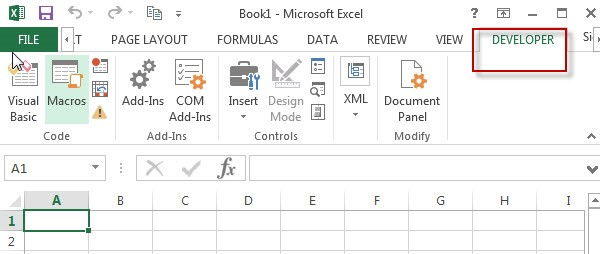## Extract all Partial Matches

Just assume that you have a range of data that contains a text string and you want to extract the few partial matches into another separate range of cells; then you might think that it’s not a big deal; because you would prefer to manually extract the few partial matches into another separate range of cells without any need of the formula;

Then congratulations because you are thinking right, but let me add up that it would be a big deal to extract the multiple partial matches into another separate range of cells and doing it manually would be a foolish attempt because you would get tired of it and would never complete your work on time.

But don’t be worry about it because after carefully reading this article, extracting multiple partial matches into another range of cells would become a piece of cake for you.

So let’s dive into the article to take you out of this fix.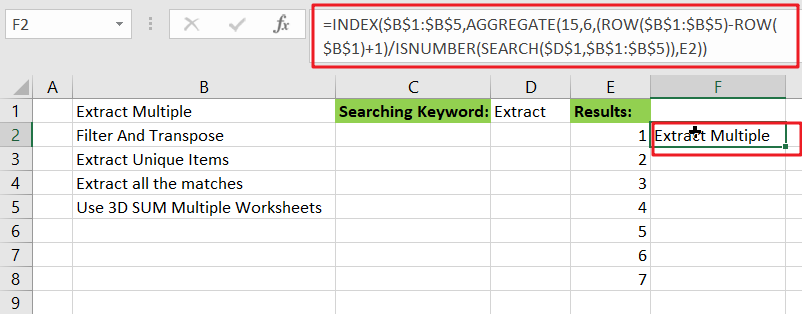## General formula

The Following formula would help you out for extracting multiple partial matches into another separate range of cells:

`=INDEX(\$B\$1:\$B\$5,AGGREGATE(15,6,(ROW(\$B\$1:\$B\$5)-ROW(\$B\$1)+1)/ISNUMBER(SEARCH(\$D\$1,\$B\$1:\$B\$5)),E2))`

## Syntax Explanations

Before going into the explanation of the formula for getting the work done efficiently, we must understand each syntax which would make it easy for you that how each syntax contributes to extracting multiple partial matches into a separate range or list of cells:

• `INDEX`: In a range or array, this index function contributes to returning the value at a given position.
• `AGGREGATE`: This function contributes to returning the aggregate result in a database or list of values in the excel sheet.
• `ROW`: In Excel, this Row function contributes toreturning the row number as a reference.
• `Absolute Reference`: The Absolute referenceis nothing but an actual fixed location in a worksheet.
• `ISNUMBER`: This function contributes to returns TRUE when a cell contains a number and FALSE if there is no number.
• `SEARCH`: This function contributes to locating the character between two text strings and returns to the number of the starting position of the first text string from the first character of the second text string.
• `Comma symbol` (,): In Excel, this comma symbol acts as a separator that helps to separate a list of values.
• `Minus Operator` (-): This minus symbol contributes to subtracting any two values.
• `Parenthesis` (): The core purpose of this Parenthesis symbol is to group the elements and to separate them from the rest of the elements.
• `Plus operator` (+): This plus operator adds the values.
• `Division` (/): This division symbol is used for dividing values or numbers.

## Let’s See How This Formula Works:

The INDEX function is the primary function having AGGREGATE, which is highly useful to figure out the matches for each row in the extracted area:

`=INDEX(\$B\$1:\$B\$5,matched_values)`

Almost all of the work is to determine and report which rows in “`\$B\$1:\$B\$5`” match the search string and report the position of each matching value to INDEX. This is accomplished by configuring the AGGREGATE function as follows:

`=AGGREGATE(15,6,(ROW(\$B\$1:\$B\$5)-ROW(\$B\$1)+1)/ISNUMBER(SEARCH(\$D\$1,\$B\$1:\$B\$5)),E2)`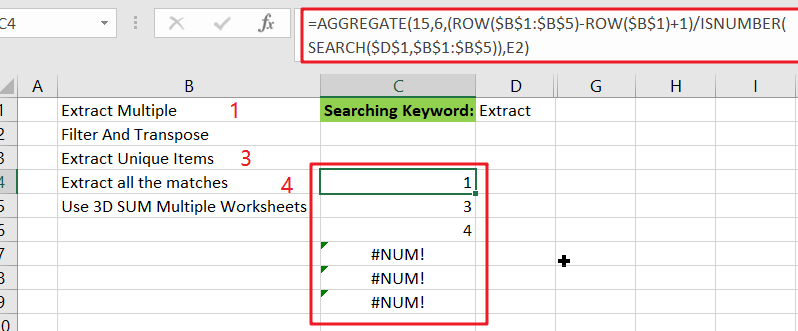The very first input or argument, which is `15`, instructs AGGREGATE to return the nth smallest values.

The second argument, `6`, specifies whether or not to ignore mistakes.

The third argument is an expression that returns an array of results that match.

The fourth input, `E2`, it specifies the “nth” value.

AGGREGATE works on arrays, and for the third argument inside AGGREGATE, the expression below builds an array :

`={(ROW(\$B\$1:\$B\$5)-ROW(\$B\$1)+1)/ISNUMBER(SEARCH(\$D\$1,\$B\$1:\$B\$5))}}`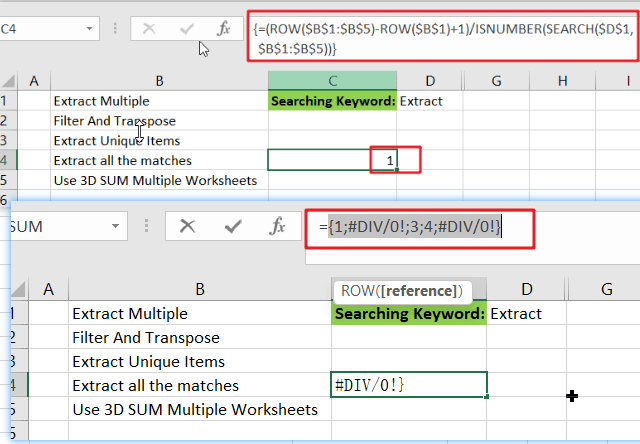In this case, the ROW function is used to build an array of relative row numbers, as that’s why SEARCH and ISNUMBER are combined to match the search string against values in the data, resulting in an array of TRUE and FALSE values.

TRUE behaves as 1 in this math operation, while FALSE behaves as 0. As a result, row numbers with a positive match are divided by 1 and survive the operation, whereas row numbers with non-matching values are destroyed and become `#DIV/0 `errors. AGGREGATE is configured to ignore errors; it ignores #DIV/0 errors and returns the “nth” smallest number from the remaining values.

## Alternative Formula with SMALL Function

You can also use an alternative formula which is based on SMALL function and IF Function to achieve the same result:

`=INDEX(\$B\$1:\$B\$5,SMALL(IF(ISNUMBER(SEARCH(\$D\$1, \$B\$1:\$B\$5)),ROW(\$B\$1:\$B\$5)-ROW(\$B\$1)+1),E2))`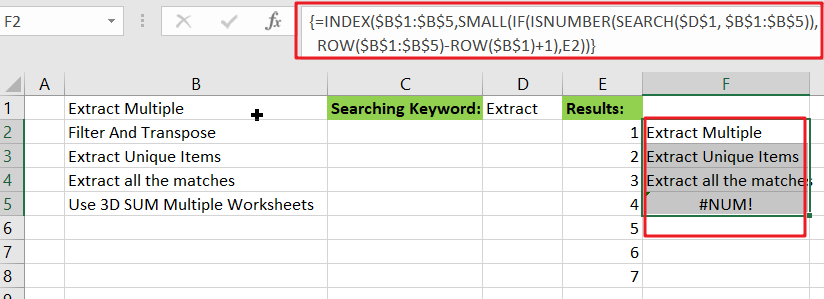Note: this is an array formula, and to get the work done, please enter it with `Control` `+` `Shift` `+` `Enter`.

### Related Functions

• Excel INDEX function
The Excel INDEX function returns a value from a table based on the index (row number and column number)The INDEX function is a build-in function in Microsoft Excel and it is categorized as a Lookup and Reference Function.The syntax of the INDEX function is as below:= INDEX (array, row_num,[column_num])…
• Excel ROW function
The Excel ROW function returns the row number of a cell reference.The ROW function is a build-in function in Microsoft Excel and it is categorized as a Lookup and Reference Function.The syntax of the ROW function is as below:= ROW ([reference])….
• Excel SMALL function
The Excel SMALL function returns the smallest numeric value from the numbers that you provided. Or returns the smallest value in the array.The syntax of the SMALL function is as below:=SMALL(array,nth) …
• Excel IF function
The Excel IF function perform a logical test to return one value if the condition is TRUE and return another value if the condition is FALSE. The IF function is a build-in function in Microsoft Excel and it is categorized as a Logical Function.The syntax of the IF function is as below:= IF (condition, [true_value], [false_value])….
• Excel ISNUMBER function
The Excel ISNUMBER function returns TRUE if the value in a cell is a numeric value, otherwise it will return FALSE.The syntax of the ISNUMBER function is as below:= ISNUMBER (value)…
• Excel SEARCH function
The Excel SEARCH function returns the number of the starting location of a substring in a text string.The syntax of the SEARCH function is as below:= SEARCH  (find_text, within_text,[start_num])…
• Excel AGGREGATE function
The Excel AGGREGATE function returns an aggregate in a list or database and ignore errors or hidden rows. The syntax of the AGGREGATE function is as below:= AGGREGATE(function_num, options, ref1,[ref2])…

## How to Sum for Cell Contains Formula Only in Excel

Sometimes values are created by formulas in cells. If we want to sum values which are created by formulas from a range, but some values which are hardcoded also list in the same range, how can we filter out matched data and only sum the filtered values correctly? By the way, if we want to sum cells that contains formulas, how can we do? Actually, we can apply ISFORMULA function to help us to solve our problem, it can return true of false if call contains a formula or not.

In this article, we will show you the method of “SUM for Cells that Contains Formula” with the application of ISFORMULA and SUMPRODUCT functions. We will create a simple formula which consists of the two functions.

We will provide a very simple instance in this article, and we will show you the syntax, argument and the usage of ISFORMULA and SUMPRODUCT functions. We will let you know how the formula works step by step clearly. After reading the article, you may know that in which situations we can choose SUMPRODUCT function to sum data.

## EXAMPLE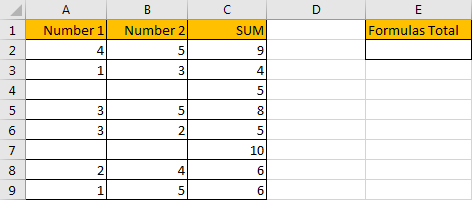In above table, we list the sum of two numbers in SUM column. But for some other numbers in the same column, they are hardcoded for example 5 in C4 and 10 in C7. In E column, we want to sum numbers which are created by formula. If we want to sum numbers ignore the hardcoded numbers, we need the help of ISFORMULA function, it can distinguish if number is generated by a formula or not. Then after filtering numbers, we can apply SUMPRODUCT function to sum filtered numbers.

## FORMULA – APPLY SUMPRODUCT FUNCTION

Step 1: In E2, enter the formula

`=SUMPRODUCT(C2:C9*ISFORMULA(C2:C9))`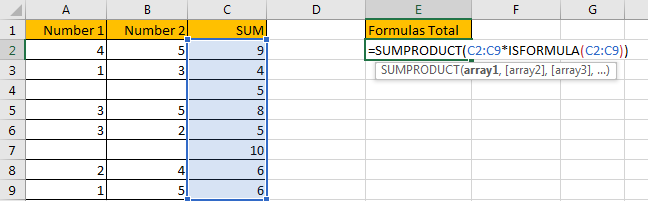Step 2: Press Enter after typing the formula.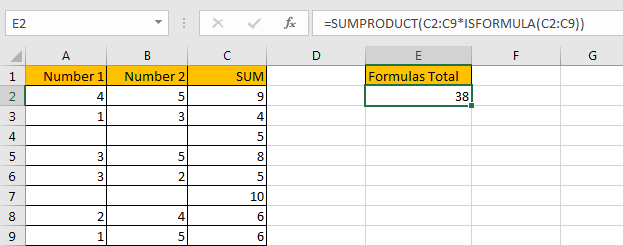We can see that the result is 38, it equals to 9+4+8+5+6+6=38. The formula works correctly.

## FUNCTION INTRODUCTION

SUMPRODUCT function can be seen as SUM+PRODUCT.

For SUMPRODUCT function, the syntax is:

`=SUMPRODUCT(array1,array2,array3, ...)`

For example, enter =SUMPRODUCT({1,2},{2,3}) in any cell, the we can get value 8, it equals to 1*2+2*3=8. You can also enter =SUMPRODUCT({1,2}*{2,3}), you can get the same result. The argument array can be a real array {1,2,3} for example or a range reference like C2:C7.

If there is only array in the formula, SUMPRODUCT will sum the numbers in the array.

Besides, SUMPRODUCT function allows entering texts, logical operators and expressions like (product=cap), texts should be enclosed into ().

ISFORMULA function can return True if cell contains a formula, or if a number is created by a formula; if returns False, that means this cell doesn’t contain any formula.

For ISFORMULA function, the syntax is:

`=ISFORMULA (reference)`

## ARGUMENTS EXPLAINATION

In this formula =SUMPRODUCT(C2:C9*ISFORMULA(C2:C9)), we applied two functions.

For ISFORMULA function, reference is C2:C9.

For SUMPRODUCT function, there is only one array argument, it is C2:C9*ISFORMULA(C2:C9).

## HOW THIS FORMULA WORKS

After explaining argument in the formula, let’s start to execute this formula from inside to outside.

First, in the formula bar, we execute ISFORMULA part. Select ISFORMULA(C2:C9), press F9 to get result, we found that an array is returned. True and False are saved in this array.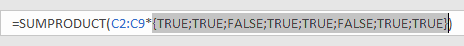Then select C2:C9 in the formula bar, press F9. We can see that values in C2:C9 are expanded in this array.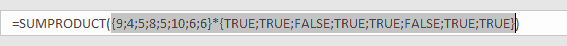Actually, in excel, if the two arrays are multiplied by each other, TRUE will be forced to convert to 1 and FALSE will be converted to 0 in math operation. So, {TRUE;TRUE;FALSE;TRUE;TRUE;FALSE;TRUE;TRUE} will be converted to {1,1,0,1,1,0,1,1} in next step of calculation. At last, this formula can be seen as

`=SUMPRODUCT({9;4;5;8;5;10;6;6}*{1,1,0,1,1,0,1,1}).`

As SUMPRODUCT function can sum products from arrays. That’s why in this case we apply SUMPRODUCT function instead of other sum related functions.

Continue executing the formula. Select {9;4;5;8;5;10;6;6}*{TRUE;TRUE;FALSE;TRUE;TRUE;FALSE;TRUE;TRUE} in the formula bar, press F9. Let’s see if products from above two arrays are displayed.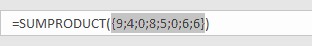As we can see, products are saved a new array {9;4;0;8;5;0;6;6}.

Select =SUMPRODUCT({9;4;0;8;5;0;6;6}) in the formula bar, press F9, 38 is displayed in formula bar. 38 is the final result.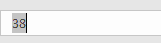1. In this instance, if we want to sum cells which doesn’t contain any cell, we can add NOT before ISFORMULA

Enter the follow formula in Cell E2:

` =SUMPRODUCT(C2:C9*NOT(ISFORMULA(C2:C9)))`

We can get result 15.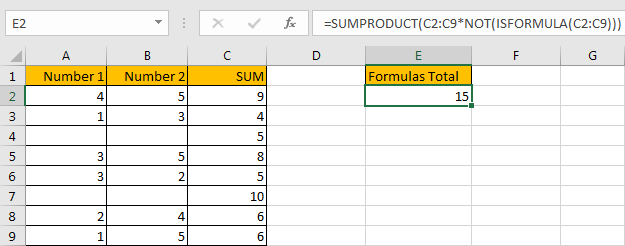NOT function here can return the opposite of the given formula ISFORMULA(C2:C9).

2. In this case we can also use SUM function to replace SUMPRODUCT function. But SUMPRODUCT function can handle arrays directly without entering Ctrl + Shift + Enter, so we often apply SUMPRODUCT function in similar situations.

After applying SUM function, press Ctrl + Shift + Enter simultaneously, we can also get correct result 38.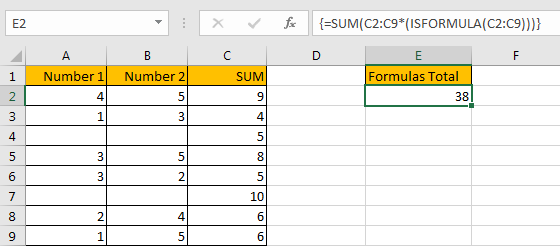## SUMMARY

1. SUMPRODUCT function can handle multiple arrays, and it sum up the products.

2. SUMPRODUCT function allows user defined range, wildcards, logical operators, expressions.

3. ISFORMULA function can return True/False if cell contains a formula or not.

4. NOT function can return the opposite of a given formula or logical operation in excel.

## Related Functions

• Excel SUMPRODUCT function
The Excel SUMPRODUCT function multiplies corresponding components in the given one or more arrays or ranges, and returns the sum of those products.The syntax of the SUMPRODUCT function is as below:= SUMPRODUCT (array1,[array2],…)…
• Excel SUM function
The Excel SUM function will adds all numbers in a range of cells and returns the sum of these values. You can add individual values, cell references or ranges in excel.The syntax of the SUM function is as below:= SUM(number1,[number2],…)
• Excel NOT function
The Excel NOT function returns the opposite of a given logical or Boolean value. For example, if you supplied with the value TRUE, the NOT function will return FALSE; If you supplied with the value FALSE, and the NOT function will TRUE. The syntax of the NOT function is as below:=NOT(logical)…

## How to Count Cells that Contain X or Y in Excel

This post will guide you how to count the number of cells that contain X or Y in a given range cells using a formula in Excel 2013/2016.You can easily to count cells equal to a specific value or text string through COUNTIF function. But if there is an easy way to count cells contain either one value or another value in a selected range of cells in Excel.

In the previous post, we talked that how to count cells that equal to either x or y in a given range with two COUNTIF functions. And it will double count cells that contain both X and Y. So it may be not your expected result, and you may only want to add it once while cells that contain both X or Y. the below steps will teach you how to count cells that contain either X or Y(do not double count).

## Count Cells Contain X or Y

Assuming that you want to count cells that contain value “excel”or “word” defined in a selected range(A1:B6).In this case, you can use the SUMPRODUCT function combining with ISNUMBER function and FIND function to count cells which are contain either  X or Y.

Enter the following formula in a blank cell, and press Enter key:

=SUMPRODUCT(–((ISNUMBER(FIND(“excel”,A1:B6)) +  ISNUMBER(FIND(“word”,A1:B6)))>0))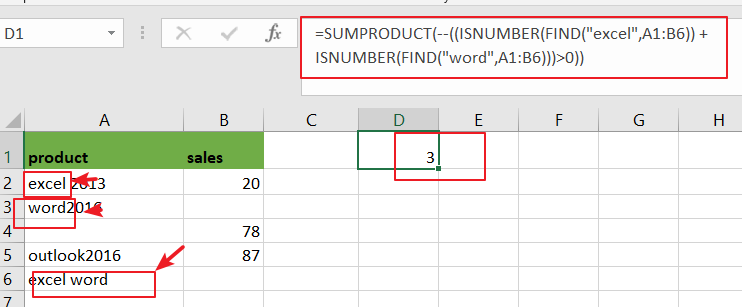Note: A2:A6 is the data range that you want to use. And both“excel” and “word” are the text values that you want to count in range A2:A6. You need to change them as you need.

NOW LET’S SEE HOW THIS FORMULA WORKS:

=FIND(“excel”,A1:B6)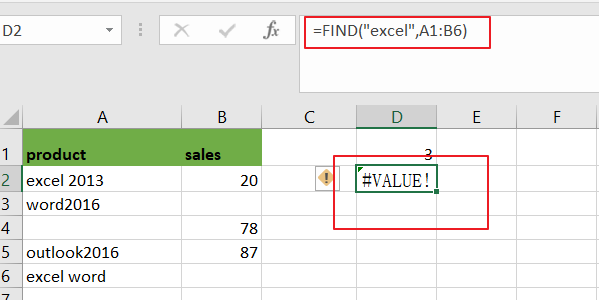The FIND function can be used to find the position of text “excel” or “word” in range A1:B6, and it returns a array result contain `1` or `#VALUE` error. When the text “excel” or “word” is not found, returns a `#VALUE` error. The returned results for the above formula is like below:

={#VALUE!,#VALUE!;1,#VALUE!;#VALUE!,#VALUE!;#VALUE!,#VALUE!;#VALUE!,#VALUE!;1,#VALUE!}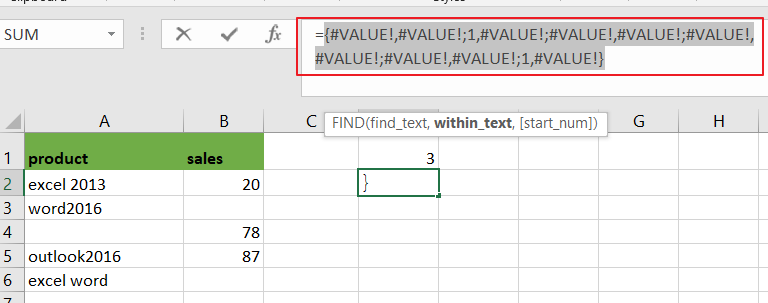You can see that there are two “`1`” value, it means that there are two cells that contain “excel”text string.

=ISNUMBER(FIND(“excel”,A1:B6))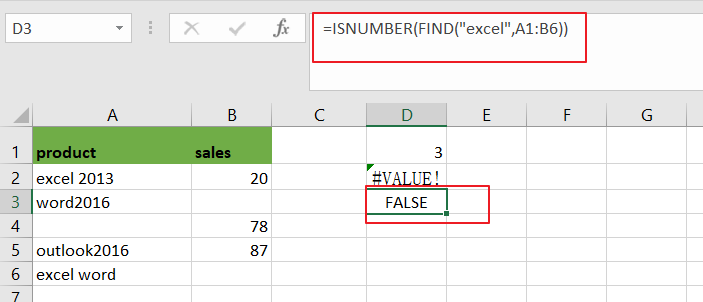Then you can use the ISNUMBER function to check the above array result, it will convert all numeric value as True, and convert other values to False.

={FALSE,FALSE;TRUE,FALSE;FALSE,FALSE;FALSE,FALSE;FALSE,FALSE;TRUE,FALSE}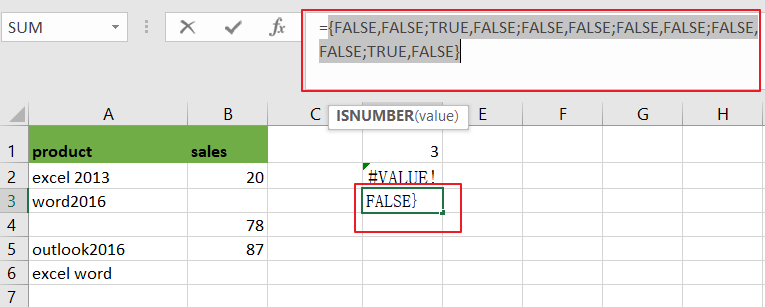You still need to convert the above logic values to number “`1`” or “`0`” with double negative character like below:

=–ISNUMBER(FIND(“excel”,A1:B6))

={0,0;1,0;0,0;0,0;0,0;1,0}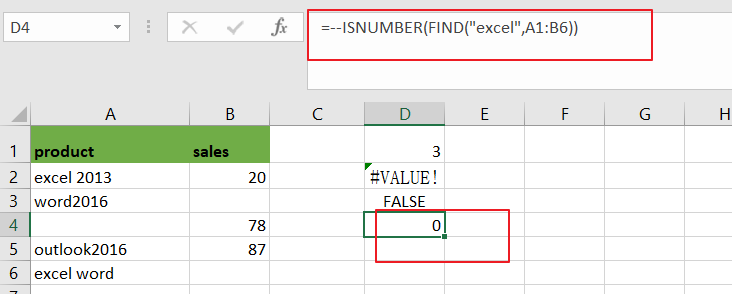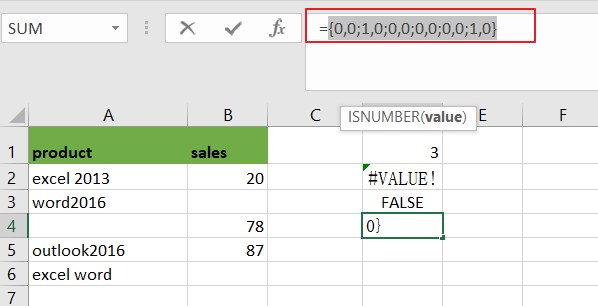Note: You can press “Fn” + “F9” to display the array result for the above array formula.

### Related Functions

• Excel SUMPRODUCT function
The Excel SUMPRODUCT function multiplies corresponding components in the given one or more arrays or ranges, and returns the sum of those products. The syntax of the SUMPRODUCT function is as below:= SUMPRODUCT (array1,[array2],…)…
• Excel COUNTIF function
The Excel COUNTIF function will count the number of cells in a range that meet a given criteria. This function can be used to count the different kinds of cells with number, date, text values, blank, non-blanks, or containing specific characters.etc.= COUNTIF (range, criteria)…
• Excel Find function
The Excel FIND function returns the position of the first text string (substring) from the first character of the second text string.The FIND function is a build-in function in Microsoft Excel and it is categorized as a Text Function.The syntax of the FIND function is as below:= FIND  (find_text, within_text,[start_num])…
• Excel ISNUMBER function
The Excel ISNUMBER function returns TRUE if the value in a cell is a numeric value, otherwise it will return FALSE.The syntax of the ISNUMBER function is as below:= ISNUMBER (value)…

## How to Highlight Cell if Same Value Exists in Another Column in Excel

This post will guide you how to highlight cell if same value exists in another column in Excel. How do I highlight cell if value is present in any cell in another column in Excel 2013/2016.

## Highlight Cell If Same Value Exists in Another Column

Assuming that you have a list of data in range A1:B5, you want to highlight cell in Column A if the value is found in Column B, how to accomplish it. You can use the Conditional Formatting feature to highlight cell in Excel. Just do the following steps:

Step1: select your range of cells in which you wish to highlight.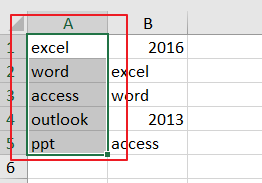Step2: go to Home tab, and click Conditional Formatting command under Styles group, and select new Rule from the context menu list. and the New Formatting Rule dialog will open.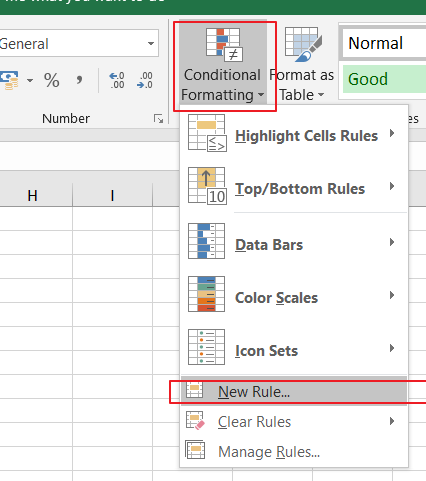Step3: click Use a formula to determine which cells to format option in the Select a Rule Type list, and type the following formula into the Format values where this formula is true text box.

=NOT(ISNA(VLOOKUP(A1,\$B:\$B,1,FALSE)))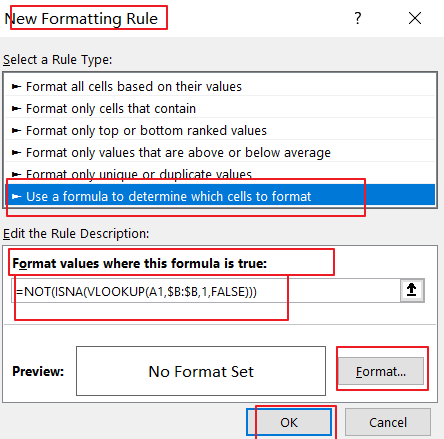Note: the Cell A1 is the first cell of the column that you want to highlight, and \$B:\$B is another column that you want to be checked)

Step4: click Format button in the New Formatting Rule dialog box, and the Format Cell dialog will open.

Step5: switch to Fill tab in the Format Cell dialog box, and choose one color as you need. click Ok button back to the New Formatting Rule dialog box.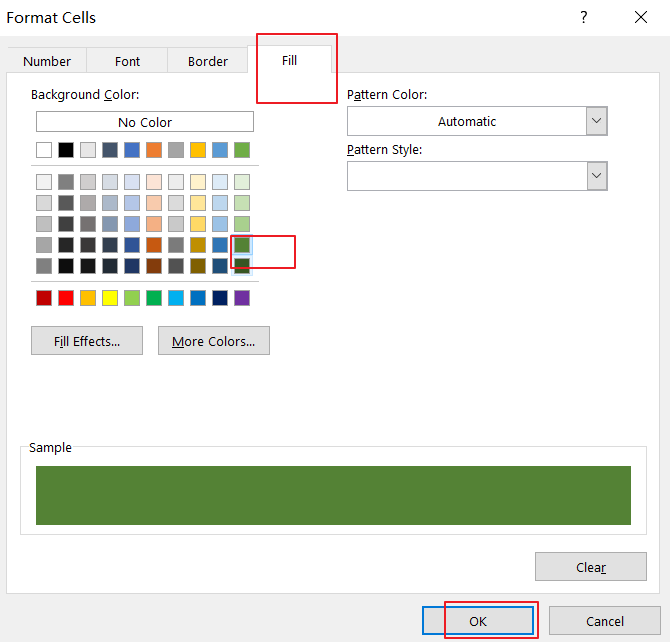Step6: click OK button. You would see that the cells in Column A have been highlighted if those values can be found in column B.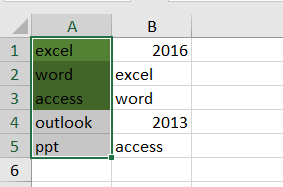## Highlight Cell If Same Value Exists in Another Column Using VBA

You can also use an Excel VBA Macro to highlight cell if value is present in another column. Just do the following steps:

Step1: open your excel workbook and then click on “Visual Basic” command under DEVELOPER Tab, or just press “ALT+F11” shortcut.Step2: then the “Visual Basic Editor” window will appear.

Step3: click “Insert” ->”Module” to create a new module.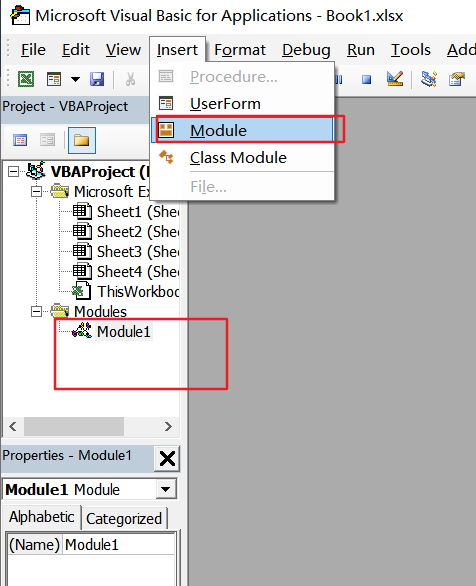Step4: paste the below VBA code  into the code window. Then clicking “Save” button.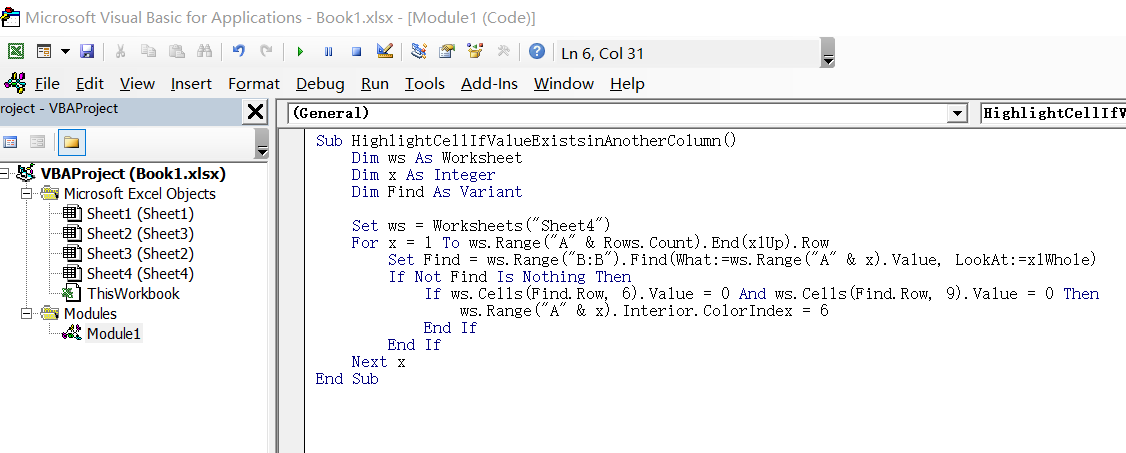```Sub HighlightCellIfValueExistsinAnotherColumn()
Dim ws As Worksheet
Dim x As Integer
Dim Find As Variant
Set ws = Worksheets("Sheet4")
For x = 1 To ws.Range("A" & Rows.Count).End(xlUp).Row
Set Find = ws.Range("B:B").Find(What:=ws.Range("A" & x).Value, LookAt:=xlWhole)
If Not Find Is Nothing Then
If ws.Cells(Find.Row, 6).Value = 0 And ws.Cells(Find.Row, 9).Value = 0 Then
ws.Range("A" & x).Interior.ColorIndex = 6
End If
End If
Next x
End Sub```

Step5: back to the current worksheet, then run the above excel macro. Click Run button.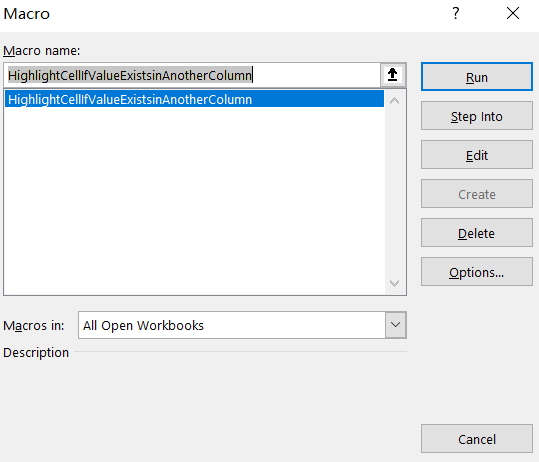Step6: let’s see the result: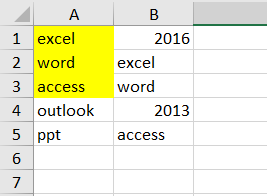### Related Functions

• Excel VLOOKUP function
The Excel VLOOKUP function lookup a value in the first column of the table and return the value in the same row based on index_num position.The syntax of the VLOOKUP function is as below:= VLOOKUP (lookup_value, table_array, column_index_num,[range_lookup])….
• Excel ISNA function
The Excel ISNA function used to check if a cell contains the #N/A error, if so, returns TRUE; otherwise, the ISNA function returns FALSE.The syntax of the ISNA function is as below:=ISNA(value)….

## Excel Ribbon

Contents:

The Ribbon is the strip of buttons and icons located above the work area. and it is organized into various tabs, such as: File, Home,Insert, Page Layout, Formulas, Data, Review, and View. while clicking on a tab, you will saw all of program’s features and commands.

This section will guide you how to customize/collapse/show/hide/reset/the Ribbon. you will also learn that how to add developer tab to ribbon and how to set quick access toolbar on ribbon.

## Ribbon Tabs

The following tabs are shown on the ribbon by default: File, Home, Insert, Page, Layout, Formulas, Data, Review and view. you will find the most basic and frequent features on Home tab.

The Home tab is the default tab in Microsoft excel.  The below screenshot shows an example of the Ribbon Tabs and Home tab in excel 2013.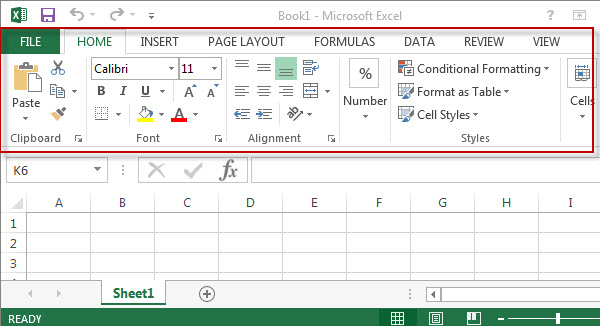## Show or Hide the Ribbon(Maximize or Minimize the Ribbon)

If you want to get more space on the screen, you can easily minimize or hide the ribbon by the following 4 ways:

#1 -To minimize the ribbon, just double-click on any of the tab names

#2 – Just press “Ctrl +F1” to hide or show the ribbon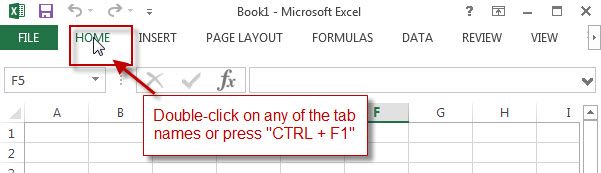#3 – Right-click on any ribbon tab name, check “Collapse the Ribbon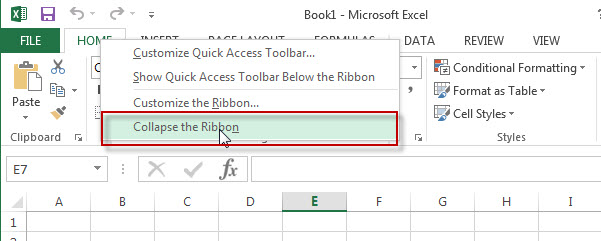#4 – Click “Ribbon Display Options” icon on the top-right corner, then click “show Tabs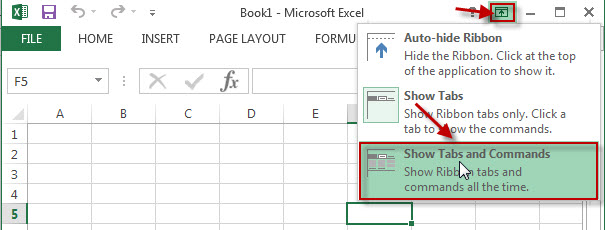Let’s see the below minimized ribbon: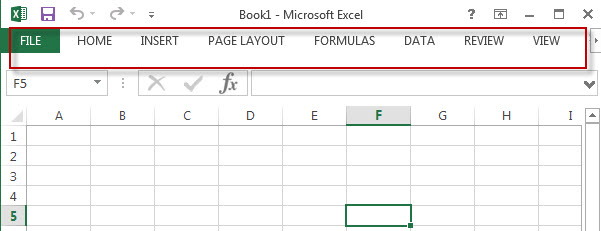Conversely, To maximize the ribbon, also following the below 4 ways:

#1 – Double-click on any of the tab names(now the ribbon is minimized)

#2 – Press “Ctrl +F1” to show the ribbon again#3 – Right-click on any ribbon tab name or anywere on the ribbon , un-check “Collapse the Ribbon#4 – Click “Ribbon Display Options” icon on the top-right corner, then click “show Tabs and Commands## Customize The Ribbon

You can easily customize the ribbon in excel , such as : add, remove, rename, reorder, reset ribbon tabs, groups, commands.

#### 1. Add Ribbon tab and groups

Step1# Right-click on any ribbon tab name or anywhere on the Ribbon , then click “Customize the Ribbon”.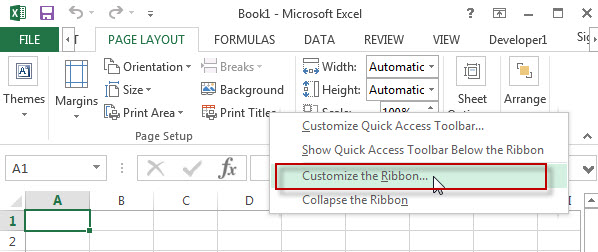Step2#  Click “New Tab” to Add a newly ribbon tab and custom ribbon group.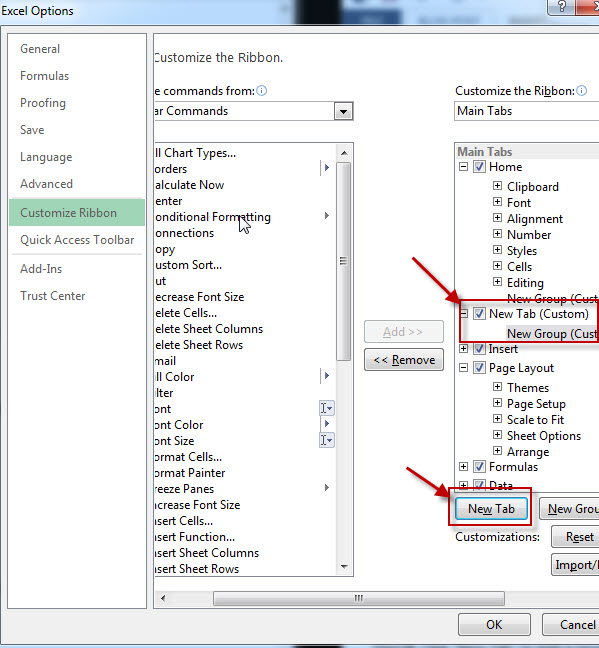#### 2. Rename ribbon tab

Step 1#  click the tab you want to rename ribbon tab(default or custom tab or groups)

Step 2#  then click “Rename

Step 3#  type the name you want to set.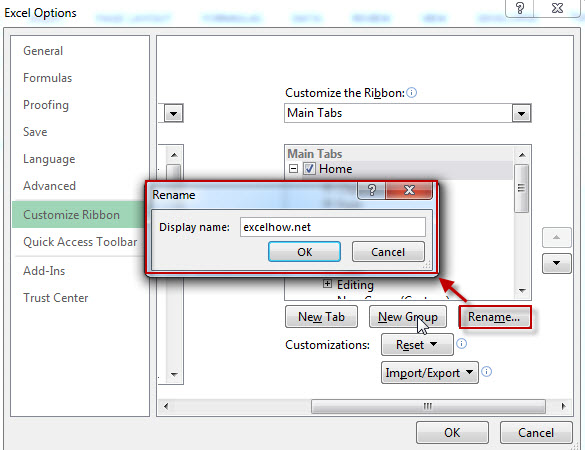#### 3. Add Ribbon command to custom group

Step 1# click the custom group that you want to add a command

Step 2# then choose one command from the left command list

Step 3# next to click “Add” command.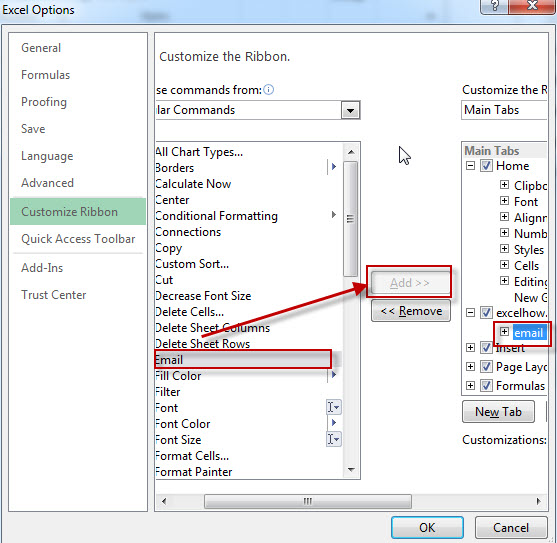#### 4. Reset ribbon tabs to default

You can reset all Customizations or just reset only selected ribbon tab,  just click “Reset” button then click  “reset all customizations” or “reset only selected ribbon tab” .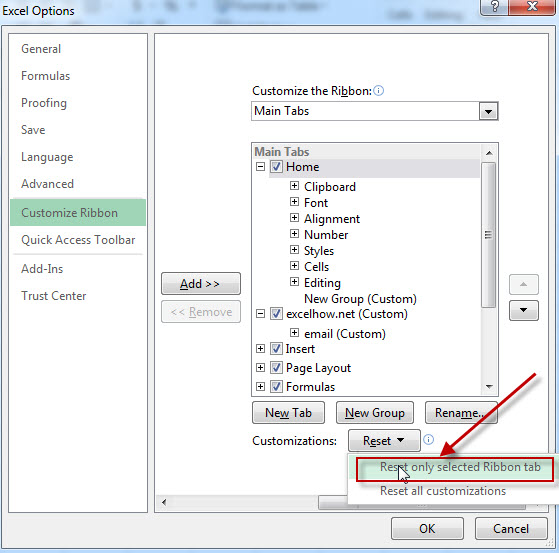The below screenshot is the customized result for ribbon tab.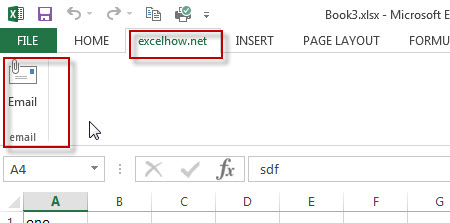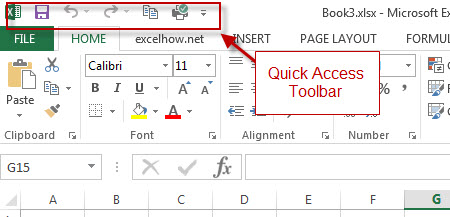Step 1#  Right-click the appropriate tab or group or everywhere place on ribbon, then click “Add to Quick Access Toolbar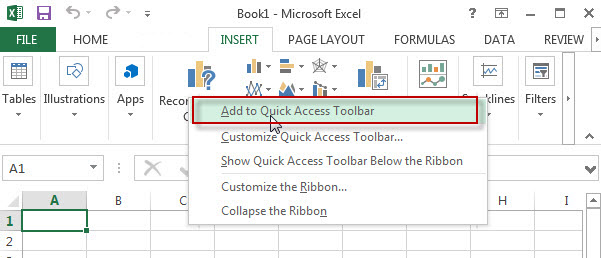How to add a command to the Quick Access Toolbar that isn’t on the ribbon? See the below steps: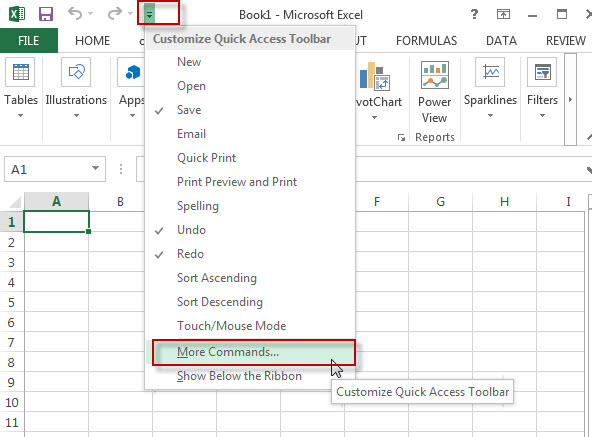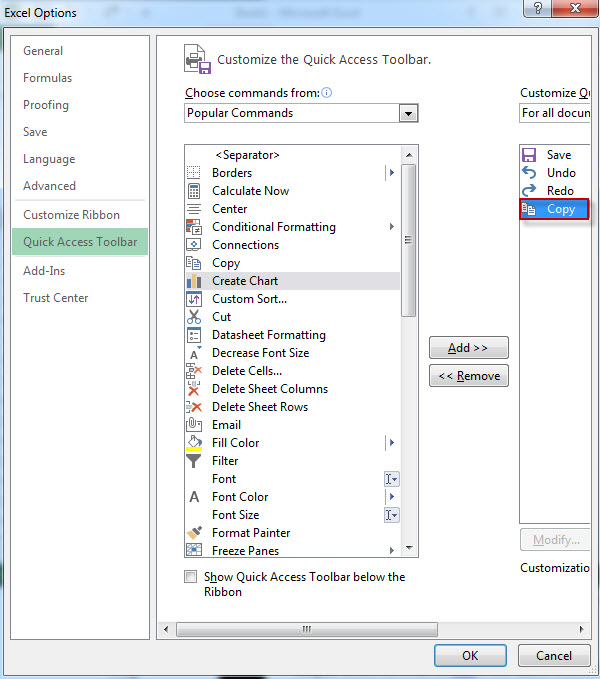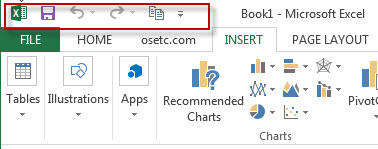## Add developer tab to ribbon

If you want to write macros, use XML commands/ActiveX controls… you need to add developer tab to ribbon, As the Developer tab is not displayed by default. To display developer Tab , just refer to the following steps:

Step 1#  Right-click any ribbon tabs or black space on the ribbon, then click “Customize the Ribbon…”Step 2# check “Developer” tabs under “Customize the ribbon” list. Then click “ok” button.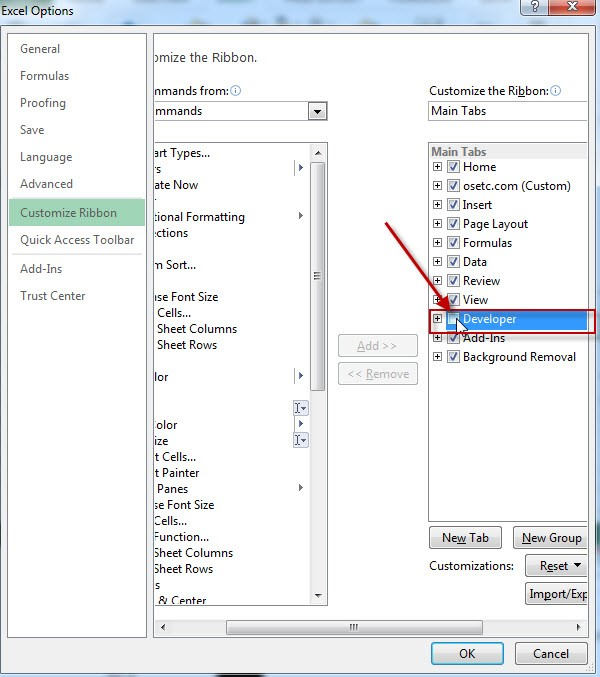Now let’s see the result: